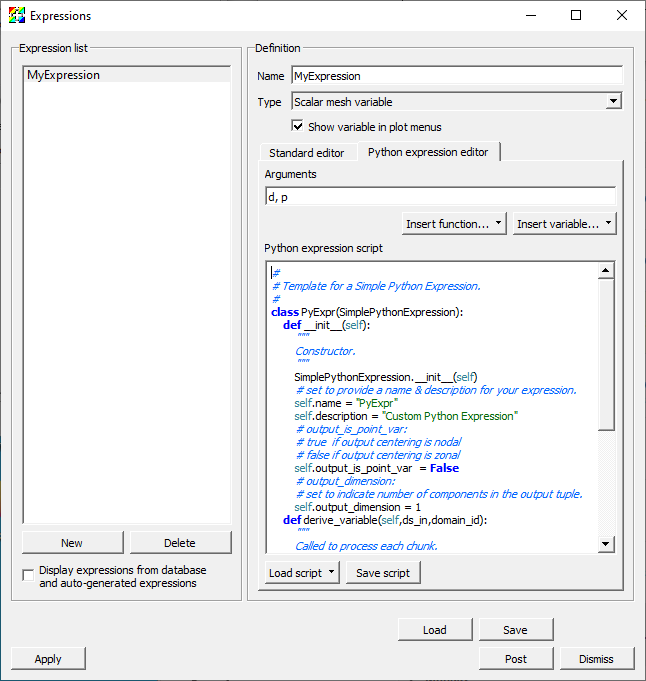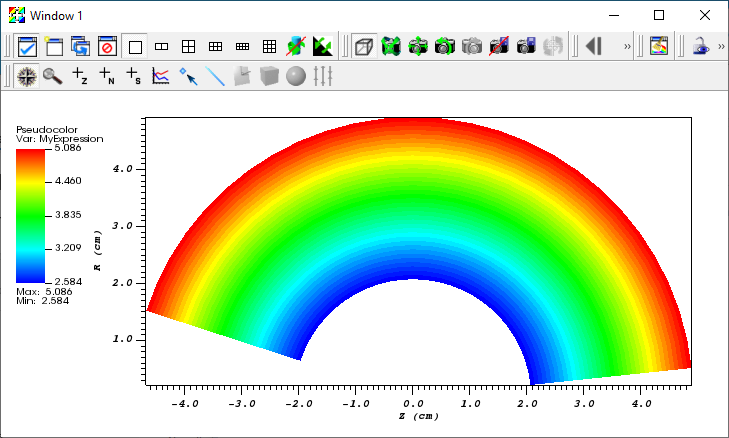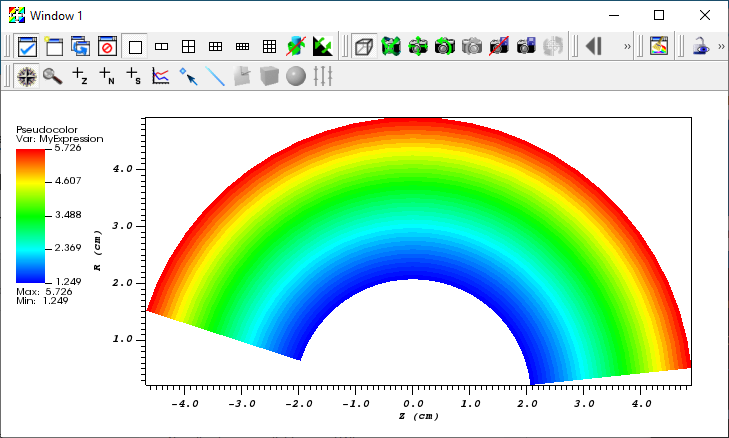# 11. Python Expressions¶

This tutorial describes using Python expressions. Python expressions are an advanced feature and should olny be used if the needed functionality is not available in the standard expression system.

## 11.1. Python Expressions Overview¶

When the available expressions fail to provide needed functionality, users can extend VisIt’s expression system in arbitrary ways using Python expressions. However, Python expressions require users to become somewhat converscent in detailed aspects of how mesh and variable data is represented in terms of VTK objects as well as how they are decomposed and processed in parallel. Nonetheless, Python expressions provide a powerful approach to filling in gaps in needed functionality.

Python expressions are run on the compute engine and will run in parallel whenever a) the compute engine is parallel and b) the input dataset is decomposed for parallel. Python expressions operate on vtkDataSets, which provide access to mesh variables as well as the mesh coordinates and topology. Python expression also have access to VisIt’s metadata as well as access to MPI when running in parallel. Python expressions return a vtkDataArray, which allows returning new variables. It is not possible to change the mesh topology or coordinates within a Python expression. However, it is possible to combine Python expressions with Cross Mesh Field Evaluation (CMFE) functions which can have the effect of changing mesh topology and coordinates. The functionality is available through the GUI and the CLI. When using the GUI, they can be created in the Expressions window. When using the CLI, they can be created with the DefinePythonExpression function.

## 11.2. Creating a Python Expression with the GUI¶

We will now go through the steps required to create a Python expression using the GUI.

Let us start by opening a file and creating a plot.

1. Open the file curv2d.silo.

2. Create a Psuedocolor plot of d.

Now let us go ahead and create the Python expression.

1. Go to Controls->Expressions.

2. This brings up the Expressions window.

3. Click New in the Expression list to create a new expression.

4. Change the Name in the Definition section to “MyExpression”.

5. Click on the Python expression editor tab in the Definition section.

6. Select Insert variable…->Scalars->d to add d to the Arguments text field.

7. Select Insert variable…->Scalars->p to add p to the Arguments text field. Note that the variable names are seperated by a comma. If the variable names are not separated by commas you will get a cryptic error message when you try to plot the expression.

8. Click Load script->Template->Simple filter to add a template of a Python expression in the Python expression script editor.Fig. 11.10 The Expressions window with the simple filter template

At this point you modify the template to create your expression. A common practice is to make modifications to the script and test it using the Pseudocolor plot. Changes to the script can be made either by modifying the script in the Python expression script editor or modifying it in an external text editor and then reloading the script. Generaly speaking, modifying the script in the Python expression script editor is easier than doing it in an external text editor except that it is difficult to tell how many spaces are at the beginning of the line since the editor does not use a fixed width font.

### 11.2.1. Developing the script in the Python expression script editor¶

The following steps can be used to iteratively develop your script using the Python expression script editor.

1. Edit the script.

2. Click the Apply button.

3. Go to Variables->d to change the variable to d. The first time you try your script this will not be necessary since the variable is already d.

4. Go to Variables->MyExpression to change the variable to “MyExpression” and execute the script.

### 11.2.2. Developing the script in a text editor¶

The following steps can be used to iteratively develop your script using a text editor.

Before you can modify the script you will need to save it.

1. Click Save script to save the script.

Now you are ready to modify the script.

1. Edit the script with your favorit editor.

3. Click the Apply button.

4. Go to Variables->d to change the variable to d. The first time you try your script this will not be necessary since the variable is already d.

5. Go to Variables->MyExpression to change the variable to “MyExpression” and execute the script.

## 11.3. Python Expression Example 1¶

This example adds two cell centered variables. It demonstrates accessing multiple variables and performing simple operations with them to generate a result.

Here is the example script.

class MyExpression(SimplePythonExpression):
def __init__(self):
SimplePythonExpression.__init__(self)
self.name = "PythonExpression"
self.description = "Add two scalar variables together"
self.output_is_point_var  = False
self.output_dimension = 1
def modify_contract(self,contract):
pass
def derive_variable(self,ds_in,domain_id):
# ds_in is the input data set
# Get the data array for the first variable
cell_vals1 = ds_in.GetCellData().GetArray(self.input_var_names)
# Get the data array for the second variable
cell_vals2 = ds_in.GetCellData().GetArray(self.input_var_names)
# Get the number of values in the variables
ncells = ds_in.GetNumberOfCells()
# Create a scalar float array with ncells values for the result
res = vtk.vtkFloatArray()
res.SetNumberOfComponents(1)
res.SetNumberOfTuples(ncells)
for i in range(ncells):
# Add the i'th value from the first variable to the i'th
# value for the second variable
val = cell_vals1.GetTuple1(i) + cell_vals2.GetTuple1(i)
# Store the value in the i'th value in the result
res.SetTuple1(i, val)
return res

py_filter = MyExpression


Let us start off by creating a Pseudocolor plot from the expression.

1. Copy the script into the Python expression script editor.

2. Click the Apply button.

3. Go to Variables->MyExpression to change the variable to the expression.Fig. 11.11 The Pseudocolor plot of MyExpression

Now let us take a look at the script and see what each portion does.

The __init__ method provides information about the expression, including

• That it inherits from SimplePythonExpression.

• The name of the expression.

• A description of the expression.

• A flag indicating that the output is not a point centered value.

• A flag that the output is a scalar.

    def __init__(self):
SimplePythonExpression.__init__(self)
self.name = "PythonExpression"
self.description = "Add two scalar variables together"
self.output_is_point_var  = False
self.output_dimension = 1


The modify_contract method can be used to request special information for the expression from VisIt. In this case it is a no-op.

    def modify_contract(self,contract):
pass


The derive_variable method performs the real work of the expression. It is passed the input vtkDataSet and the domain_id.

    def derive_variable(self,ds_in,domain_id):
# ds_in is the input data set


The following lines get the vtkDataArrays for the cell values for the two variables and the number of cells.

        # Get the data array for the first variable
cell_vals1 = ds_in.GetCellData().GetArray(self.input_var_names)
# Get the data array for the second variable
cell_vals2 = ds_in.GetCellData().GetArray(self.input_var_names)
# Get the number of values in the variables
ncells = ds_in.GetNumberOfCells()


The following lines set the output vtkDataArray to be an array of floats with 1 component and ncells values.

        # Create a scalar float array with ncells values for the result
res = vtk.vtkFloatArray()
res.SetNumberOfComponents(1)
res.SetNumberOfTuples(ncells)


Now we loop over the cells, setting the output value for each cell.

        for i in range(ncells):


The following lines add the two variables for the current cell.

            # Add the i'th value from the first variable to the i'th
# value for the second variable
val = cell_vals1.GetTuple1(i) + cell_vals2.GetTuple1(i)


The following lines set the result value for the current cell.

            # Store the value in the i'th value in the result
res.SetTuple1(i, val)


Once we have finished processing all the cells, we return the vtkDataArray.

        return res


### 11.3.1. Using your Python Expression with the CLI¶

The Python expression we just created can also be used with the CLI.

We will start by saving the script we just created.

1. Click Save script and save the script with the name MyExpression.py.

The following script will open curv2d.silo and create a Pseudocolor plot of the expression.

OpenDatabase("/usr/gapps/visit/data/curv2d.silo")

DefinePythonExpression("MyExpression", ['d', 'p'], file="MyExpression.py")

DrawPlots()


## 11.4. Python Expression Example 2¶

This example operates on 2D meshes and takes the distance around the edges of each cell and multiplies it by the value of the cell. It demonstrates accessing the coordinates and topology of the mesh as well as a variable.

Here is the example script.

from math import sqrt
class MyExpression(SimplePythonExpression):
def __init__(self):
SimplePythonExpression.__init__(self)
self.name = "PythonExpression"
self.description = "Multiply the variable by sum of cell edge lengths in 2D"
self.output_is_point_var  = False
self.output_dimension = 1
def modify_contract(self,contract):
pass
def derive_variable(self,ds_in,domain_id):
# ds_in is the input data set
# Get the data array for the variable
cell_vals = ds_in.GetCellData().GetArray(self.input_var_names)
# Get the number of values in the variable
ncells = ds_in.GetNumberOfCells()
# Create a scalar float array with ncells values for the result
res = vtk.vtkFloatArray()
res.SetNumberOfComponents(1)
res.SetNumberOfTuples(ncells)
for i in range(ncells):
# Get the i'th cell
cell = ds_in.GetCell(i)
# Get the number of edges in the cell
nedges = cell.GetNumberOfEdges()
# Sum up the lengths of the edges
sum = 0.
for j in range(nedges):
# Get the j'th edge
edge = cell.GetEdge(j)
# Calculate the edge length from the end points
pt1 = ds_in.GetPoint(edge.GetPointId(0))
pt2 = ds_in.GetPoint(edge.GetPointId(1))
len = sqrt((pt2 - pt1) * (pt2 - pt1) +
(pt2 - pt1) * (pt2 - pt1) +
(pt2 - pt1) * (pt2 - pt1))
sum = sum + len
# Multiply the sum by the i'th value of the variable
sum *= cell_vals.GetTuple1(i)
# Store the value in the i'th value in the result
res.SetTuple1(i, sum)
return res

py_filter = MyExpression


Let us start off by creating a Pseudocolor plot from the expression.

1. Copy the script into the Python expression script editor.

2. Click the Apply button.

3. Go to Variables->MyExpression to change the variable to the expression.Fig. 11.12 The Pseudocolor plot of MyExpression

The __init__ and modify_contract methods are the same as the previous example, so we will only look at the derive_variable method.

    def derive_variable(self,ds_in,domain_id):
# ds_in is the input data set


The following lines get the vtkDataArray for the cell values and the number of cells.

        # Get the data array for the variable
cell_vals = ds_in.GetCellData().GetArray(self.input_var_names)
# Get the number of values in the variable
ncells = ds_in.GetNumberOfCells()


The following lines set the output vtkDataArray to be an array of floats with 1 component and ncells values.

        # Create a scalar float array with ncells values for the result
res = vtk.vtkFloatArray()
res.SetNumberOfComponents(1)
res.SetNumberOfTuples(ncells)


Now we loop over the cells, setting the output value for each cell.

        for i in range(ncells):


The following lines get the current cell and the number of edges in the cell.

            # Get the i'th cell
cell = ds_in.GetCell(i)
# Get the number of edges in the cell
nedges = cell.GetNumberOfEdges()


Now we loop over the edges, calculating the sum of the lengths of the edges.

            # Sum up the lengths of the edges
sum = 0.
for j in range(nedges):


We calculate the length of the edge from the 3D coordinates of the end points of the edge, which we add to the sum.

                # Get the j'th edge
edge = cell.GetEdge(j)
# Calculate the edge length from the end points
pt1 = ds_in.GetPoint(edge.GetPointId(0))
pt2 = ds_in.GetPoint(edge.GetPointId(1))
len = sqrt((pt2 - pt1) * (pt2 - pt1) +
(pt2 - pt1) * (pt2 - pt1) +
(pt2 - pt1) * (pt2 - pt1))
sum = sum + len


Once we have summed the lengths of the edges we multiply the sum by the cell value and set it in the result.

            # Multiply the sum by the i'th value of the variable
sum *= cell_vals.GetTuple1(i)
# Store the value in the i'th value in the result
res.SetTuple1(i, sum)


Once we have finished processing all the cells, we return the vtkDataArray.

        return res


### 11.4.1. Using your Python Expression with the CLI¶

This Python expression can also be used with the CLI, just as the one in the first example, except the specification of the variables to use is slightly different. Since you are only passing a single variable you would use ("d") for the list of variables.

OpenDatabase("/usr/gapps/visit/data/curv2d.silo")

DefinePythonExpression("MyExpression", ("d"), file="MyExpression.py")